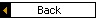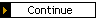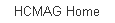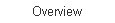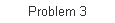Sub-problem 2b - Page 1 of 3 ID# C202B01

Sub-problem 2b: Moe Road PM peak hour - With Conditions

Lane Utilization
Another issue that we can address at this intersection is lane utilization. When the lane use isn’t balanced, i.e., there isn’t equal traffic in each lane, the performance analysis needs to account for that fact.

At the Moe Road intersection, this situation exists for the westbound approach. The two lanes merge into one about 250 feet downstream of the intersection (click here to see a photo). The right-hand lane ends and its traffic must merge left. This creates a tendency for drivers to use the innermost lane, especially when the flows are light to moderate. The day we collected data, 57% of the westbound traffic was in the inner lane. At other times, that number can be as high as 75%.

From an HCM perspective, the lane utilization factor, fLU, is defined as the ratio of the average volume per lane to the volume in the heaviest-used lane. Therefore, if the total volume is 1,000 vehicles per hour and there are two lanes, the average volume per lane is 500 vph. If the heaviest-used lane sees 750 vehicles per hour, the lane utilization coefficient is 0.67 = 500/750. If the lane utilizations are given in percents, the lane utilization coefficient is given by the ratio of the average percentage of traffic per lane, in this case 50% divided by the maximum percentage per lane, or 75%.

The day we collected data, 57% of the westbound traffic was in the inner lane, so the lane utilization coefficient on that day was 0.81 = 50%/57%. We used this value in all the analyses. At other times, we indicated that the percentage of traffic in the inner lane can reach as high as 75%. We’re going to use that value for comparison purposes.

Discussion: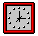Can you think of intersections where lane utilization is an issue? How have you handled it in the past? How important was it to take it into account? Have you thought about doing lane-by-lane analysis? Would that help? What about simulation? Do simulation models let you consider lane utilization?

[ Back ] to Sub-Problem 2a [ Continue ] with Sub-Problem 2b